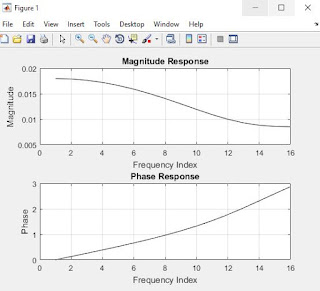Impact-Site-Verification: dbe48ff9-4514-40fe-8cc0-70131430799e

## Search This Blog

Here is the simple MATLAB code to find out N point DFT and IDFT.

You can get transfer function also using this code. Phase and Magnitude both are plotted separately.

### MATLAB Program

#### Coding:

clc;
clear all;
close all;
Length=input('Enter the length of DFT(for best result enter in terms of power of 2):');
Theta=0:pi/Length:pi;
Num=[0.05 0.033 0.008];
Den=[0.06 4 1];
Transfer=tf(Num,Den);
[Freq,W]=freqz(Num,Den,Length);
grid on;
figure(1);
subplot(2,1,1);
plot(abs(Freq),'k');
disp(abs(Freq'));
title('Magnitude Response');
xlabel('Frequency Index');
ylabel('Magnitude');
grid on;
subplot(2,1,2)
disp(angle(Freq'));
plot(angle(Freq),'k');
title('Phase Response');
xlabel('Frequency Index');
ylabel('Phase');
grid on;

#### OUTPUT

Enter the length of DFT(for best result enter in terms of power of 2):16
Columns 1 through 12
0.0180    0.0179    0.0176    0.0172    0.0166    0.0159    0.0150    0.0140    0.0130    0.0119    0.0109    0.0100
Columns 13 through 16
0.0093    0.0088    0.0086    0.0085
Columns 1 through 12
0   -0.1273   -0.2557   -0.3863   -0.5206   -0.6603   -0.8074   -0.9646   -1.1356   -1.3247   -1.5363   -1.7738
Columns 13 through 16
-2.0360   -2.3148   -2.5972   -2.8730

#### Figure:DFT/IDFT Plot